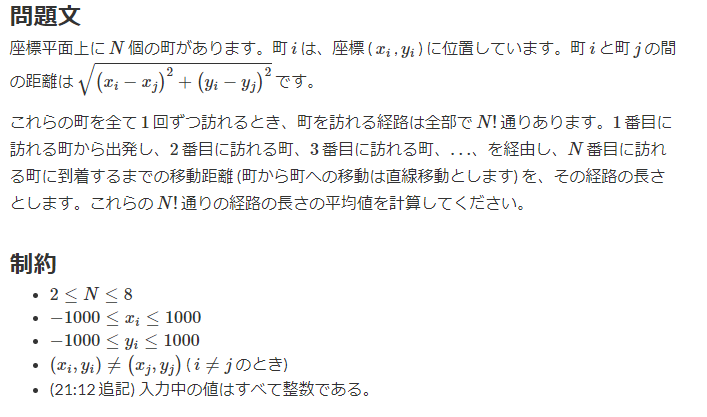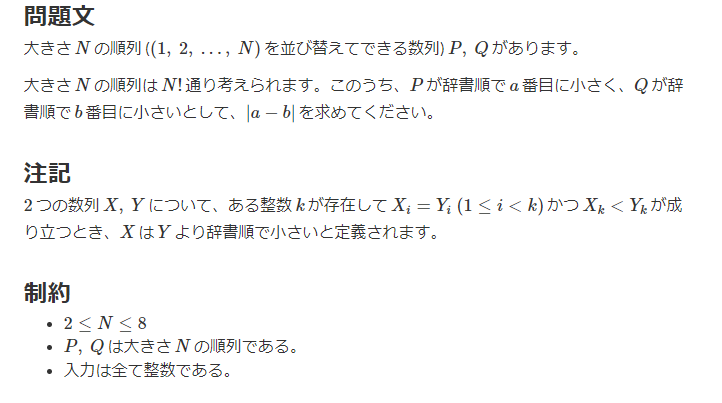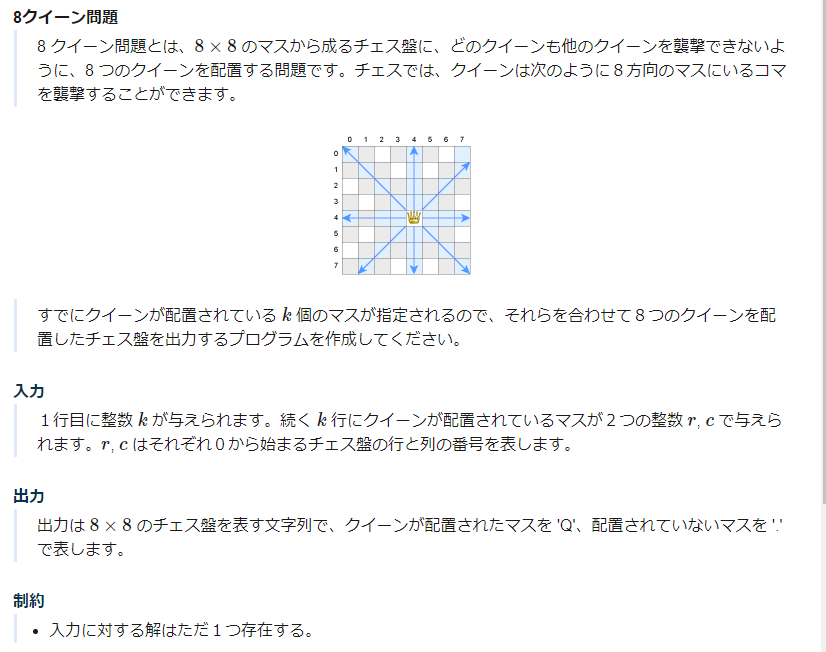# 1. Purpose

Solve 100 past questions that beginners and intermediates should solve in Python. The goal is to turn light blue by the time you finish solving everything.

# 2. Summary

Permutation problems can often be solved by using `itertools` well. `permutaions`When`combination`When`product`I use it a lot, so I learned this area by trying various sample data.

# 3. Main story

## 015 --017 Full search: Permutation full search

1. AtCoder Beginner Contest 145 C - Average Length``````
N = int(input())
towns = [tuple(map(int, input().split())) for _ in range(N)]

total_distance = 0
count = 0
for start in range(N-1):
for end in range(start+1, N):
start_x, start_y = towns[start]
end_x, end_y = towns[end]

total_distance += ((end_x - start_x)**2 + (end_y - start_y)**2)**0.5
count += 1

# factor = math.factorial(N)Then
# answer = total_distance * (factor * (N - 1) / count) /It becomes a factor and the formula can be transformed as follows
answer = total_distance * ((N - 1) / count)

``````

There are various calculation methods here. To be honest, I enumerate all the arrangements with ` permutation`, find the distance in all, and take the average. I think this will still work, but I tried it as an answer with a little less calculation. The policy is

1. Sum the distances of all two point combinations
2. The answer is this sum multiplied by `` `(N -1) / number of combinations` `.

is The above 2 is derived from the expression transformation left as a comment in the code.

1. AtCoder Beginner Contest 150 C - Count Order``````import itertools

N = int(input())
P = tuple(map(int, input().split()))
Q = tuple(map(int, input().split()))

count = 1
for p in itertools.permutations(range(1, N+1)):

if p == P:
count_P = count
if p == Q:
count_Q = count

count += 1

ans = abs(count_P - count_Q)
print(ans)

``````

Believe that python's `itertools.permutations` will list them in lexicographic order (!) I'll write it straightforwardly. The policy is

1. Enumerate permutations from 1 to N with `itertools.permutations`
2. Count the counts to enumerate and record ``count_P``` and` `count_Q``` where P and Q match.
3. The answer is the difference between the two

is. `itertools`If you don't have one, you'll have to think a little more, but it's convenient, so I used it without thinking.

### 017. ALDS_13_A-8 Queens Problem``````
import itertools
import copy

for i in range(1, 8):
if 0 <= c+i and c+i < 8:
grid[r][c+i] = -1 #right
if 0 <= c-i and c-i < 8:
grid[r][c-i] = -1 #left
if 0 <= r+i and r+i < 8:
grid[r+i][c] = -1 #under
if 0 <= r-i and r-i < 8:
grid[r-i][c] = -1 #Up
if 0 <= r-i and r-i < 8 and 0 <= c+i and c+i < 8:
if grid[r-i][c+i] == 9:
continue
grid[r-i][c+i] = -1 #Upper right
if 0 <= r+i and r+i < 8 and 0 <= c+i and c+i < 8:
if grid[r+i][c+i] == 9:
continue
grid[r+i][c+i] = -1 #Lower right
if 0 <= r+i and r+i < 8 and 0 <= c-i and c-i < 8:
if grid[r+i][c-i]  == 9:
continue
grid[r+i][c-i] = -1 #Lower left
if 0 <= r-i and r-i < 8 and 0 <= c-i and c-i < 8:
if grid[r-i][c-i] == 9:
continue
grid[r-i][c-i] = -1 #upper left

grid[r][c] = 9 #myself

return grid

if __name__ == "__main__":

k = int(input())
grid = [ * 8 for _ in range(8)]
for _ in range(k):
r, c = map(int, input().split())

# 0~Try all 7 numbers in a permutation
#Think of the generated permutations as row numbers for subscripts and column numbers for elements
for perm in itertools.permutations(range(8)):
copy_grid = copy.deepcopy(grid) #Use a new grid every time
count = 0
for row, col in enumerate(perm):
if copy_grid[row][col] == -1:
break
if copy_grid[row][col] == 9:
continue

copy_grid[row][col] = 9
count += 1

if count == 8 - k: # 8-break if you put k queens
break

for row in range(8):
for col in range(8):
if copy_grid[row][col] == -1:
print('.', end='')
else:
print('Q', end='')
print()

``````

Originally I wrote it in Numpy, but can't AOJ use Numpy? I couldn't submit it due to an error, so I rewrote it with a normal list. The policy is

1. First, set the chess board to `` `grid```, set the position where the queen is located to 9, the part where the queen can attack is -1, and the other blank parts are 0.
2. Create a function ``flag_queen_load ()`` that updates the board when a queen is placed at a certain coordinate` `(r, c)` `.
3. After that, try all the placements to determine if they can be placed successfully.
4. When trying all the ways, enumerate the permutations from 0 to 7 with ```itertools.permutations (range (8)) `` `, and think of the generated permutations as row numbers for subscripts and column numbers for elements. Masu
5. After that, if the board is -1, you can not put it, so ``break```, if it is 9, it is OK, so` `continue```
6. The answer is when you can put a new queen `` `8-k``` times

is.

The function ``` flag_queen_load ()` `` is dirty, so I should have written it a little cleaner.